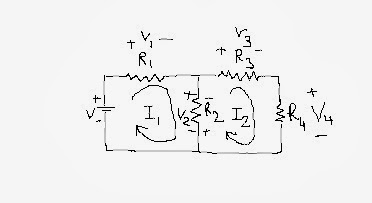### Mesh Analysis Problems ( Examples Solved)

Searching the books for your studies can be so easy
You can search in the search bar for your book .........................................................................

Mesh Analysis Problems (Examples Solved):

Mesh Analysis Problems can be understand better with the help of examples and solved problems. Mesh analysis solved problems are described here to make you feel comfortable in solving questions. Mesh analysis solved problem is given below:

In this mesh analysis problem two loops can be made. In first loop current I1 flows. Due to flow of current I1 voltage drop takes place in the resistor R1 and R2. The voltage drops are denoted by V1 and V2.
In the second loop current I2 flows and due to flow of this current voltage drop across element R3, R4 and R2 take place.Mesh Analysis Problems (Solved Example Circuit)

The direction of loop current can be taken randomly. The point at which current enters the resistor is taken as positive polarity and the point from which current leaves the resistor is considered as negative voltage polarity.

For common branch between two loops, when writing equation for loop 1, voltage drop in the common resistor is taken by the direction of flow of current in loop 1. When writing equation for loop 2 the direction of voltage drop in the common branch is taken as the direction of current in loop 2.

Because of this the direction of voltage drop in resistor R2 is taken in opposite polarities for the two loops.

Mesh Analysis Problems Equations for loops are:

Loop 1 equation is

Loop 2 equation is:

On solving these two equations, you will get the value of I1 and I2.
Mesh Analysis Problems can be solved by using the above said method.